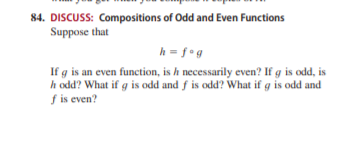# 84. DISCUSS: Compositions of Odd and Even Functions Suppose that h = f•g If g is an even function, is h necessarily even? If g is odd, is h odd? What if g is odd and f is odd? What if g is odd and f is even?

Question

. DISCUSS: Compositions of Odd and Even Functions
Suppose that
h  f  g
If g is an even function, is h necessarily even? If g is odd, is
h odd? What if g is odd and f is odd? What if g is odd and
f is even?help_outlineImage Transcriptionclose84. DISCUSS: Compositions of Odd and Even Functions Suppose that h = f•g If g is an even function, is h necessarily even? If g is odd, is h odd? What if g is odd and f is odd? What if g is odd and f is even? fullscreen

### Want to see this answer and more?

Experts are waiting 24/7 to provide step-by-step solutions in as fast as 30 minutes!*

*Response times may vary by subject and question complexity. Median response time is 34 minutes for paid subscribers and may be longer for promotional offers.
Tagged in
Math
Algebra

### Other